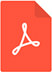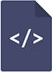Home Magazines Editors-in-Chief FAQs Contact Us

# Dynamics, stability and sustainable optimal control in wolf-moose systemsPDF Full Text

# Abstract

A wolf-moose predator-prey system is defined as a nonlinear continuous time differential equation system. The parameters are estimated via a discretized approximation and empirical data from Isle Royale, USA, representing a 61 year period. All parameter estimates confirm the hypotheses and all P-values are below 5%. Possible dynamic equilibria are determined as explicit general functions of the parameters and as numerical values based on the empirical data. General dynamic properties of the system are determined via phase -plane analysis and nonlinear simulation. The nonlinear system is also linearized close to the single equilibrium with two strictly positive populations. The explicit equations of the time path of the linearized system are compared to the nonlinear simulation. Both methods give almost identical solutions close to the equilibrium. Far from the equilibrium, the time paths of the two methods deviate considerably. The solution is a stable converging spiral (center) (unstable diverging spiral) in case the system equilibrium prey population is located at a higher (the same) (lower) level than the population level that gives maximum sustainable net production. Based on the empirically determined expected parameter values, the system is stable but converges very slowly, as a spiral, to the two-species equilibrium. The estimated standard deviations of the parameters can be used to determine the probability that the system solution is a center or an unstable diverging spiral. The optimal management of the wolf-moose system is also determined via sustainable optimal control. Moose hunting and adjustments of the wolf population based on different prices and values of sustainable population levels are derived. Optimal hunting and stock levels are determined and reported as explicit functions of all parameters and prices.

# Keywords

predator prey system, dynamics, stability, differential equation system, linearization, economic optimization, sustainable control